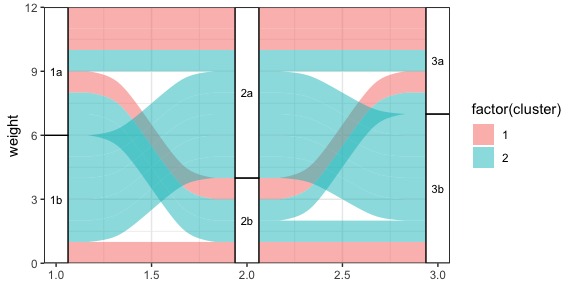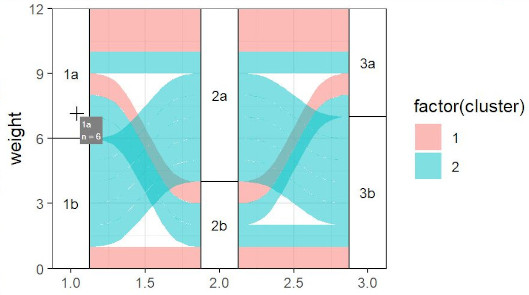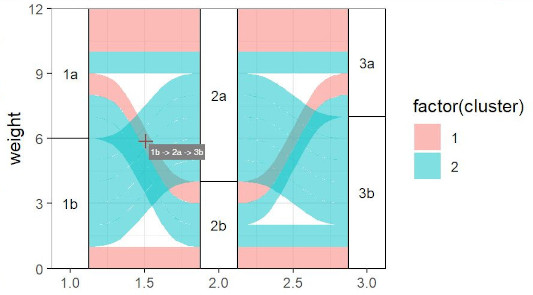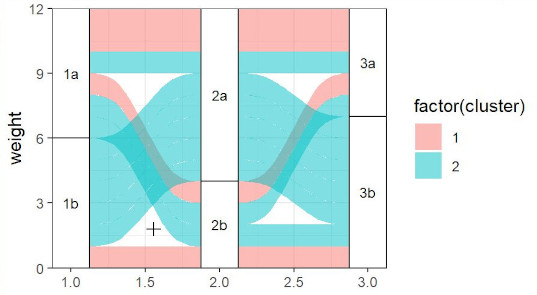# Tooltips for ggalluvial plots in Shiny apps

knitr::opts_chunkset(fig.width = 6, fig.height = 3, fig.align = "center") library(ggalluvial) ## Problem In an interactive visualization, it is visually cleaner and better for interpretation if labels and other information appear as “tooltips” when the user hovers over or clicks on elements of the plot, rather than displaying all the labels on the plot at one time. However, the ggalluvial package does not natively include this functionality. It is possible to enable this using functions from several other packages. This vignette illustrates a Shiny app that displays an alluvial plot with tooltips that appear when the user hovers over two different plot elements: strata created with geom_stratum() and alluvia created with geom_alluvium(). The tooltips that appear when the user hovers over elements of the plot show a text label and the number of flows included in each group. This is made relatively straightforward because if the user hovers or clicks somewhere inside a ggplot panel, Shiny automatically returns information about the location of the mouse cursor in plot coordinates. That means the main work we have to do is to extract or manually recalculate the coordinates of the different plot elements. With that information, we can determine which plot element the cursor is hovering over and display the appropriate information in the tooltip or other output method. Note: The app demonstrated here depends on the packages htmltools and sp, in addition of course to ggalluvial and shiny. Please be aware that all of these packages will need to be installed on the server where your Shiny app is running. ### Hovering over and clicking on strata Enabling hovering over and clicking on strata is straightforward because of their rectangular shape. We only need the minimum and maximum x and y coordinates for each of the rectangles. The rectangles are evenly spaced along the x-axis, centered on positive integers beginning with 1. The width is set in geom_stratum() so, for example, we know that the x-coordinates of the first stratum are 1 ± width/2. The y-coordinates can be determined from the number of rows in the input data multiplied by their weights. ### Hovering over and clicking on alluvia Hovering over and clicking on alluvia are more difficult because the shapes of the alluvia are more complex. The default shape of the polygons includes an xspline curve drawn using the grid package. We need to manually reconstruct the coordinates of the polygons, then use sp::pointInPolygon() to detect which, if any, polygons the cursor is over. ## Data for reproducible example This toy dataset is used for the example app. example_data <- data.frame( weight = rep(1, 12), ID = 1:12, cluster = rep(c(1, 2), c(4, 8)), grp1 = rep(c('1a', '1b', '1a', '1b'), c(3, 2, 3, 4)), grp2 = rep(c('2a', '2b', '2a', '2b', '2a'), c(2, 2, 2, 2, 4)), grp3 = rep(c('3a','3b', '3a', '3b'), c(3, 2, 2, 5)) ) Here is a static plot generated using the toy dataset. ggplot(example_data, aes(y = weight, axis1 = grp1, axis2 = grp2, axis3 = grp3)) + geom_alluvium(aes(fill = factor(cluster)), knot.pos = 0.25) + geom_stratum(width = 1/8, reverse = TRUE) + geom_text(aes(label = after_stat(stratum)), stat = "stratum", reverse = TRUE, size = rel(3)) + theme_bw() + scale_x_continuous(expand = c(0, 0)) + scale_y_continuous(expand = c(0, 0))## Structure of the example app Here, we will go over each section of the code in detail. The full code is reproduced at the bottom of this document. ### User interface The app includes a minimal user interface with two output elements. ui <- fluidPage( fluidRow(tagsdiv(
style = "position: relative;",
plotOutput("alluvial_plot", height = "500px",
hover = hoverOpts(id = "plot_hover")
),
htmlOutput("tooltip")))
)

The elements are:

• a plotOutput with the argument hover defined, to enable behavior determined by the cursor’s plot coordinates whenever the user hovers over the plot.
• an htmlOutput for the tooltip that appears next to the cursor on hover.

Both of the elements are wrapped in a fluidRow() and a div() tag.

Note: This vignette only illustrates how to display output when the user hovers over an element. If you want to display output when the user clicks on an element, the corresponding argument to plotOutput() is click = clickOpts(id = "plot_click"). This will return the location of the mouse cursor in plot coordinates when the user clicks somewhere within the plot panel.

### Server function

The server function is more complex. Its general structure looks like this, in pseudocode:

server <- function(input, output, session) {

output$alluvial_plot <- renderPlot({ '<Create "ggplot" object for alluvial plot.>' '<Build alluvial plot and assign globally.>' '<Extract data from built plot object used to create alluvium polygons.>' '<Use polygon splines to generate coordinates of alluvium boundaries.>' '<Convert coordinates from grid units to plot units and assign globally.>' '<Render the plot.>' }) output$tooltip <- renderText({
if ('<mouse cursor is within the plot panel>') {
if ('<mouse cursor is within a stratum box>') {
'<Render stratum tooltip.>'
} else {
if ('<mouse cursor is within an alluvium polygon>') {
'<Render alluvium tooltip.>'
}
}
}
})

}

First, we create the ggplot object for the alluvial plot, then we call the ggplot_build() function to build the plot without displaying it. The next lines of code are to “reverse engineer” the polygon coordinates. Finally, we call renderPlot() to pass the plot to output.

Next, we define the tooltip with a renderText() expression. Within that expression, we first extract the cursor’s plot coordinates from the user input. We determine whether the cursor is hovering over a stratum and if so, display the appropriate tooltip.screenshot of tooltip on stratum

If the mouse cursor is not hovering over a stratum, we determine whether it is hovering over an alluvium polygon and if so, display different information in the tooltip.screenshot of tooltip on alluvium

If the mouse cursor is hovering over an empty region of the plot, nothing is returned by renderText() and so no tooltip text box is displayed.screenshot of cursor over empty region

Let’s take a deeper dive into each part of the server function.

#### 1. Drawing plot and extracting coordinates

The first part of the server function includes code to draw the plot and build it with ggplot_build(). Note that the global assignment operator <<- is used to assign node_width and pbuilt so they are both accessible outside the renderPlot() expression.

Note: In the example presented here, strictly speaking all of the plot drawing and coordinate extracting code could be outside the server() function, because the plot itself does not change with user input. However if you are building an app where the plot changes in response to user input, for example a menu of options of which variables to display, the plot drawing code has to be inside the renderPlot() expression. So we’ve left it there in the example code.

output$alluvial_plot <- renderPlot({ # Width of node boxes node_width <<- 1/4 p <- ggplot(example_data, aes(y = weight, axis1 = grp1, axis2 = grp2, axis3 = grp3)) + geom_alluvium(aes(fill = factor(cluster)), knot.pos = 0.25) + geom_stratum(width = node_width, reverse = TRUE) + geom_text(aes(label = after_stat(stratum)), stat = "stratum", reverse = TRUE, size = rel(3)) + theme_bw() + scale_x_continuous(expand = c(0, 0)) + scale_y_continuous(expand = c(0, 0)) # Build the plot. Use global assignment so that this object is accessible # later. pbuilt <<- ggplot_build(p) Now for the hard part: reverse-engineering the coordinates of the alluvia polygons. This makes use of pbuilt$data[], a data frame with the individual elements of the alluvial plot. We add an additional column for width, which has a value of 1/3 hard-coded into ggalluvial::geom_alluvium(), then split the data frame by group (groups correspond to the individual alluvium polygons). We apply the unexported function ggalluvial:::data_to_xspline() to each element of the list to get the x-spline coordinates. Then, we pass the x-spline coordinates to the function grid::xsplineGrob() to convert them into a grid object. We pass the resulting object to grid::xsplinePoints(). At this point we now have the coordinates of the alluvium polygons.

  # Use built plot data to recalculate the locations of the flow polygons:

# Add width parameter, and then convert built plot data to xsplines
data_draw <- transform(pbuilt$data[], width = 1/3) groups_to_draw <- split(data_draw, data_draw$group)
group_xsplines <- lapply(groups_to_draw,
ggalluvial:::data_to_xspline,
knot.prop = TRUE)

# Convert xspline coordinates to grid object.
xspline_coords <- lapply(
group_xsplines,
function(coords) grid::xsplineGrob(x=coords$x, y=coords$y,
shape=coords$shape, open=FALSE) ) # Use grid::xsplinePoints to draw the curve for each polygon xspline_points <- lapply(xspline_coords, grid::xsplinePoints) The coordinates we have are in grid plotting units but we need to convert them into the same units as the axes on the plot. We do this by determining the range of the x and y axes in grid units (xrange_old and yrange_old), then fixing the range of the x axis as 1 to the number of strata, adjusted by the width of the nodes, and the y axis to the number of rows in the data (again, this is possible here because each flow polygon is exactly 1 unit high). We define a function new_range_transform() inline and apply it to each set of coordinates, assigning the resulting object globally so it can be accessed later. Now we have the coordinates of the polygons in plot units! So we can close the expression after returning the plot.  # Define the x and y axis limits in grid coordinates (old) and plot # coordinates (new) xrange_old <- range(unlist(lapply( xspline_points, function(pts) as.numeric(pts$x)
)))
yrange_old <- range(unlist(lapply(
xspline_points, function(pts) as.numeric(pts$y) ))) xrange_new <- c(1 - 1/6, 3 + 1/6) yrange_new <- c(0, nrow(example_data)) # Define function to convert grid graphics coordinates to data coordinates new_range_transform <- function(x_old, range_old, range_new) { (x_old - range_old)/(range_old - range_old) * (range_new - range_new) + range_new } # Using the x and y limits, convert the grid coordinates into plot # coordinates. Use global assignment. polygon_coords <<- lapply(xspline_points, function(pts) { x_trans <- new_range_transform(x_old = as.numeric(pts$x),
range_old = xrange_old,
range_new = xrange_new)
y_trans <- new_range_transform(x_old = as.numeric(pts$y), range_old = yrange_old, range_new = yrange_new) list(x = x_trans, y = y_trans) }) # Return plot p }, res = 200) #### 2. Logic for determining cursor location and displaying tooltips First, we check whether the cursor is inside the plot panel. If it is not, the element plot_hover of the input will be NULL. output$tooltip <- renderText(
if(!is.null(input$plot_hover)) { ... } ... ) Next, we check whether the cursor is over a stratum. We round the x-coordinate of the mouse cursor in data units to the nearest integer, then determine whether the x-coordinate is within node_width/2 of that integer. If so, the mouse cursor is horizontally within the box. hover <- input$plot_hover
x_coord <- round(hover$x) if(abs(hover$x - x_coord) < (node_width / 2)) { ... }

The nearest integer to the y-coordinate corresponds to the row of the data frame because we set reverse = TRUE and all weight = 1 in the input data. So, for example, the first row of the data frame corresponds to y range c(0, 1), the second c(1, 2), and so forth. This gives us all the information we need to find the index of the rows of the input data that goes with the stratum the cursor is on. Note: It is necessary for the input data to be sorted in ascending order of the group column, named cluster in this example. If it is not sorted in this way, the relative order of the flows along the y-axis will not correspond to their order in the data.

node_row <-
pbuilt$data[]$x == x_coord & hover$y > pbuilt$data[]$ymin & hover$y < pbuilt$data[]$ymax

We get the name of the stratum as well as the total number of flows passing through it.

node_label <- pbuilt$data[]$stratum[node_row]
node_n <- pbuilt$data[]$n[node_row]

Finally, we render a tooltip using the div tag and passing it to htmltools::renderTags(). Note that the tooltip positioning is provided in CSS coordinates (pixels), not data coordinates. This does not require any additional effort on our part because plot_hover also includes the mouse cursor location in those units.

renderTags(
tags$div( node_label, tags$br(),
"n =", node_n,
style = paste0(
"position: absolute; ",
"top: ", hover$coords_css$y + offset, "px; ",
"left: ", hover$coords_css$x + offset, "px; ",
"background: gray; ",
"color: white; "
)
)
)$html If the cursor is not over a stratum, the next logic checks whether it is over an alluvium. This is done using the function sp::point.in.polygon applied across each of the polygons for which we defined the coordinates inside the renderPlot expression. hover_within_flow <- sapply( polygon_coords, function(pol) point.in.polygon(point.x = hover$x,
point.y = hover$y, pol.x = pol$x,
pol.y = pol$y) ) If at least one polygon is beneath the mouse cursor, we locate the corresponding row in the input data and extract information to display in the tooltip. In the situation where there are more than one polygon overlapping, we get the information for the polygon that is plotted last by calling rev() on the logical vector returned by point.in.polygon(). This means that the tooltip will display information from the alluvium that appears “on top” in the plot. In this example, we will display the names of all the nodes that the alluvium passes through. coord_id <- rev(which(hover_within_flow == 1)) flow_id <- example_data$ID[coord_id]
axis_values <- example_data[flow_id, c('grp1', 'grp2', 'grp3')]

We render a tooltip that shows the names of all the nodes that the hovered path passes through, using very similar syntax to the above tooltip.

renderTags(
tags$div( paste(axis_values, collapse = ' -> '), style = paste0( "position: absolute; ", "top: ", hover$coords_css$y + offset, "px; ", "left: ", hover$coords_css$x + offset, "px; ", "background: gray; ", "padding: 3px; ", "color: white; " ) ) )$html

## Conclusion

This vignette demonstrates how to enable tooltips for ggalluvial plots in Shiny apps. However it’s important to note that some of the workarounds are slightly inelegant. This may not be the optimal way to do it — other solutions are certainly possible!

## Appendix

### Complete app code

library(ggalluvial)
library(shiny)
library(htmltools)
library(sp)

example_data <- data.frame(
weight = rep(1, 12),
ID = 1:12,
cluster = rep(c(1, 2), c(4, 8)),
grp1 = rep(c('1a', '1b', '1a', '1b'), c(3, 2, 3, 4)),
grp2 = rep(c('2a', '2b', '2a', '2b', '2a'), c(2, 2, 2, 2, 4)),
grp3 = rep(c('3a','3b', '3a', '3b'), c(3, 2, 2, 5))
)

# User interface
ui <- fluidPage(
fluidRow(tags$div( style = "position: relative;", plotOutput("alluvial_plot", height = "500px", hover = hoverOpts(id = "plot_hover") ), htmlOutput("tooltip"))) ) server <- function(input, output, session) { # Draw plot and extract coordinates output$alluvial_plot <- renderPlot({

# Width of node boxes
node_width <<- 1/4

p <- ggplot(example_data,
aes(y = weight, axis1 = grp1, axis2 = grp2, axis3 = grp3)) +
geom_alluvium(aes(fill = factor(cluster)), knot.pos = 0.25) +
geom_stratum(width = node_width, reverse = TRUE) +
geom_text(aes(label = after_stat(stratum)),
stat = "stratum",
reverse = TRUE,
size = rel(3)) +
theme_bw() +
scale_x_continuous(expand = c(0, 0)) +
scale_y_continuous(expand = c(0, 0))

# Build the plot. Use global assignment so that this object is accessible
# later.
pbuilt <<- ggplot_build(p)

# Use built plot data to recalculate the locations of the flow polygons:

# Add width parameter, and then convert built plot data to xsplines
data_draw <- transform(pbuilt$data[], width = 1/3) groups_to_draw <- split(data_draw, data_draw$group)
group_xsplines <- lapply(groups_to_draw,
ggalluvial:::data_to_xspline,
knot.prop = TRUE)

# Convert xspline coordinates to grid object.
xspline_coords <- lapply(
group_xsplines,
function(coords) grid::xsplineGrob(x = coords$x, y = coords$y,
shape = coords$shape, open = FALSE) ) # Use grid::xsplinePoints to draw the curve for each polygon xspline_points <- lapply(xspline_coords, grid::xsplinePoints) # Define the x and y axis limits in grid coordinates (old) and plot # coordinates (new) xrange_old <- range(unlist(lapply( xspline_points, function(pts) as.numeric(pts$x)
)))
yrange_old <- range(unlist(lapply(
xspline_points,
function(pts) as.numeric(pts$y) ))) xrange_new <- c(1 - 1/6, 3 + 1/6) yrange_new <- c(0, nrow(example_data)) # Define function to convert grid graphics coordinates to data coordinates new_range_transform <- function(x_old, range_old, range_new) { (x_old - range_old)/(range_old - range_old) * (range_new - range_new) + range_new } # Using the x and y limits, convert the grid coordinates into plot # coordinates. Use global assignment. polygon_coords <<- lapply(xspline_points, function(pts) { x_trans <- new_range_transform(x_old = as.numeric(pts$x),
range_old = xrange_old,
range_new = xrange_new)
y_trans <- new_range_transform(x_old = as.numeric(pts$y), range_old = yrange_old, range_new = yrange_new) list(x = x_trans, y = y_trans) }) # Return plot p }, res = 200) output$tooltip <- renderText(
if(!is.null(input$plot_hover)) { hover <- input$plot_hover
x_coord <- round(hover$x) if(abs(hover$x - x_coord) < (node_width / 2)) {
# Display node information if cursor is over a stratum box.

# Determine stratum name from x and y coord, and the n.
node_row <- pbuilt$data[]$x == x_coord &
hover$y > pbuilt$data[]$ymin & hover$y < pbuilt$data[]$ymax
node_label <- pbuilt$data[]$stratum[node_row]
node_n <- pbuilt$data[]$n[node_row]

# Offset, in pixels, for location of tooltip relative to mouse cursor,
# in both x and y direction.
offset <- 5

# Render tooltip
renderTags(
tags$div( node_label, tags$br(),
"n =", node_n,
style = paste0(
"position: absolute; ",
"top: ", hover$coords_css$y + offset, "px; ",
"left: ", hover$coords_css$x + offset, "px; ",
"background: gray; ",
"color: white; "
)
)
)$html } else { # Display flow information if cursor is over a flow polygon: what # alluvia does it pass through? # Calculate whether coordinates of hovering cursor are inside one of the # polygons. hover_within_flow <- sapply( polygon_coords, function(pol) point.in.polygon(point.x = hover$x,
point.y = hover$y, pol.x = pol$x,
pol.y = pol$y) ) if (any(hover_within_flow)) { # Find the alluvium that is plotted on top. (last) coord_id <- rev(which(hover_within_flow == 1)) # Get the corresponding row ID from the data. flow_id <- example_data$ID[coord_id]
# Get the axis 1-3 values for all axes for that row ID.
axis_values <- example_data[flow_id, c('grp1', 'grp2', 'grp3')]

offset <- 5

# Render tooltip
renderTags(
tags$div( paste(axis_values, collapse = ' -> '), style = paste0( "position: absolute; ", "top: ", hover$coords_css$y + offset, "px; ", "left: ", hover$coords_css$x + offset, "px; ", "background: gray; ", "padding: 3px; ", "color: white; " ) ) )$html
}
}
}
)
}

shinyApp(ui = ui, server = server)